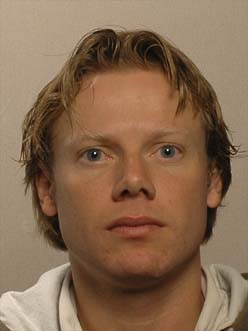# Dr. ir. Frank van der Meulen (TUD)Date: Friday 09 June 2017

Time: 12:45 - 13:30 (Lunch available from 12:35)

Room: RA 1501 (Ravelijn)

## Title: Bayesian computation for diffusion processes

Abstract:

Diffusion processes are widely used in application areas such as biology and finance. These processes are mathematically defined as solutions to a system of stochastic differential equations of the form

dXt = b(t;Xt) dt + (t;Xt)dWt; X0 = x0 2 Rd:

Here, W is a multivariate Wiener process. Suppose that the process X is discretely observed, say at times 0 = t0 < t1 < ... < tn. The statistical problem consists of inferring the unknown parameter appearing in both the drift coefficient b and diffusion coefficient o. This problem has received much attention over the past two decades and poses a very challenging problem, mainly due to the lack of a closest form expression for the likelihood of the given statistical model.
In this talk I will first position this research problem within computational statistics. Then I will discuss solutions using the Bayesian approach to statistics. I will end with some remaining challenges which are related to ongoing research.

This concerns joint work with Moritz Schauer (Leiden University), Omiros Papaspiliopoulos (Universitat Pompeu Fabra) and Harry van Zanten (University of Amsterdam).

 Schauer, M. and Van der Meulen, F. H. and Van Zanten, J. H. (2017),Guided proposals for simulating multi-dimensional diusion bridges, Bernoulli 23(4A) (2017), 2917-2950.
 Van der Meulen, F. H. and Schauer, M. (2017), Bayesian estimation of discretely observed multi-dimensional diusion processes using guided proposals, Electronic Journal of Statistics 11(1), 2358- 2396.
 F.H. van der Meulen and M.R. Schauer (2016) Bayesian estimation of incompletely observed diusions, arXiv:1606.04082.